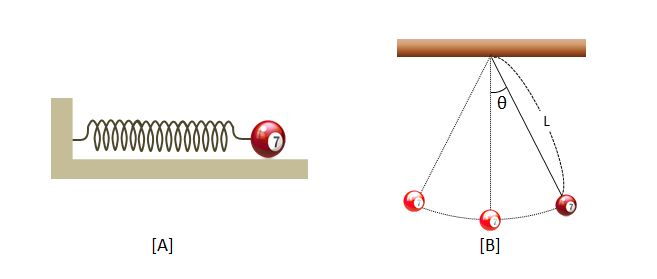# Two pendulumsIn the two pictures above, [A] is a spring pendulum installed on a frictionless floor, and [B] is a simple pendulum installed on a ceiling. Which of the following statements is correct? (Ignore all frictional forces, and suppose that the angle $\theta$ of the simple pendulum is very small.)

a) The directions of the forces acting on [A] and [B] are opposite to the directions of the displacements.

b) The greater the masses of the two pendulums, the greater the periods of the harmonic oscillations.

c) If we install these two pendulums on the moon where the gravitational acceleration is smaller than on the earth, then only the period of [B] gets longer.

×